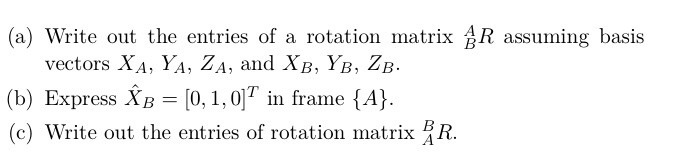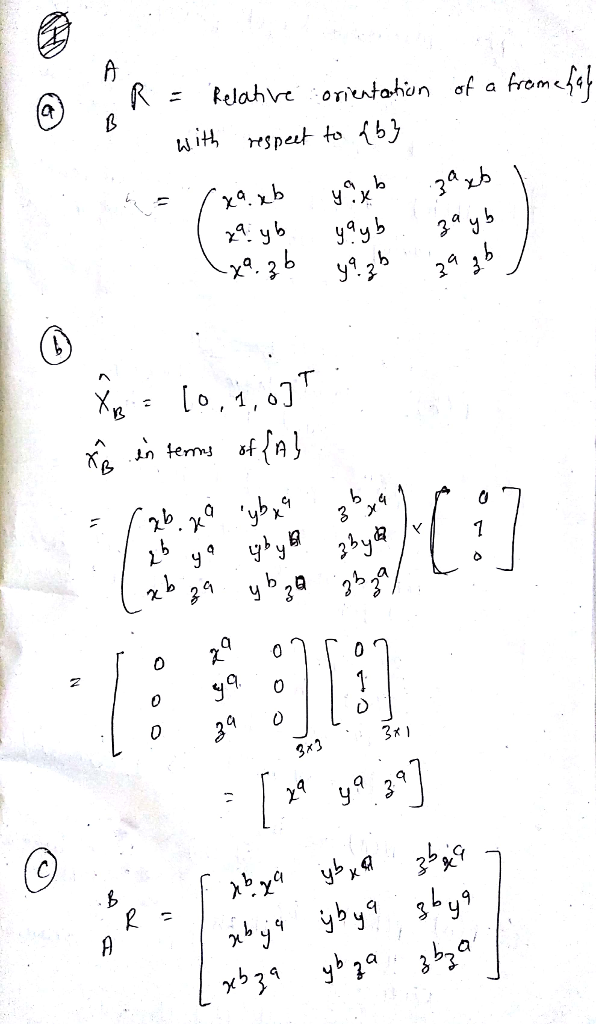# Homework Solution: robotics questions…

robotics questions(a) Write out the entries of a rotation matrix ^A _B R assuming basis vectors X_A, Y_A, Z_A, and X_B, Y_B, Z_B. (b) Express X cap_B = [0, 1, 0]^T in frame {A}. (c) Write out the entries of rotation matrix ^B _A R.